# 16 Communication with Shiny

Communication of research is central to environmental data science, and while this can use many venues such as professional meetings and publications, outreach on the internet is especially important. This chapter will delve into probably the best way to build a web site for communicating our research: Shiny, used for building interactive web apps, using interactive controls to allow the user to manipulate any of the R analyses and graphics you’ve learned about. But as we’ll see right away, these interactive controls can also be used in R Markdown.

Four ways to use Shiny:

• Shiny Web App: As a web application hosted on a server. This is probably the best way to communicate your work, and includes all of Shiny interactive methods. But to get it on the web, you’ll need to get it hosted. One option for this is to use https://www.shinyapps.io which will host 5 apps with 25 hours usage/month for free, or you can pay for more access. We’ll look at some complete Shiny Web Apps later in this chapter, but also see https://shiny.rstudio.com and https://shiny.rstudio.com/tutorial to learn more.
• Shiny app run locally: The same Shiny web app but run locally on your own computer in RStudio. When you’re building your web app, this is where you’ll start anyway, prepping your app to run well before publishing it to the web.
• Shiny Document: As an R Markdown document with the shiny runtime. It creates an HTML document, using interactive Shiny components. We’re going to start with this method, since it’s the easiest introduction to the input widgets and output options. To learn more, see Chapter 19 in R Markdown, the Definitive Guide () and https://www.rstudio.com/wp-content/uploads/2015/02/rmarkdown-cheatsheet.pdf.
• Shiny Presentation: Also using the R Markdown system, creates an IOSlides presentation which uses interactive Shiny components.

## 16.1 Shiny Document

We’ll start with creating a Shiny Document as a way to introduce Shiny interactive components, but it’s also not a bad option if you just want to create an interactive environment for your own work, and it’s also pretty easy to share with others, like we’ve already seen with R Markdown. But since Shiny requires a host to run the R code on, we can’t really create it as interactive objects in this book without accessing a hosted Shiny app (which you can do, see Xie (2021)), but we’ll include snips below to show you what it looks like. After we’ve learned about these interactive components, we can also use them in a Shiny Web App, but there will be other structural elements we’ll need to add.

To create a Shiny Document, use File>New File>R Markdown… to initiate the process, where we’ll specify that we want to create a Shiny document and give it a title. For this first one, we’ll just create its default document that accesses the faithful (Old Faithful eruptions) built-in data, so we’ll give it that name.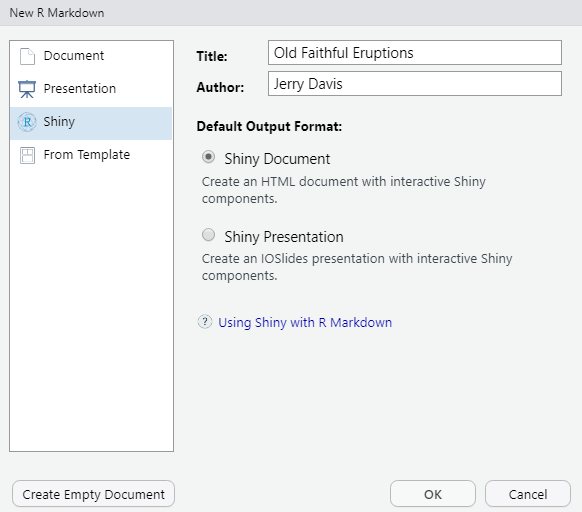Figure 16.1: New Shiny Document dialog

When we OK this, we’ll see the R Markdown document opened in the RStudio script editor.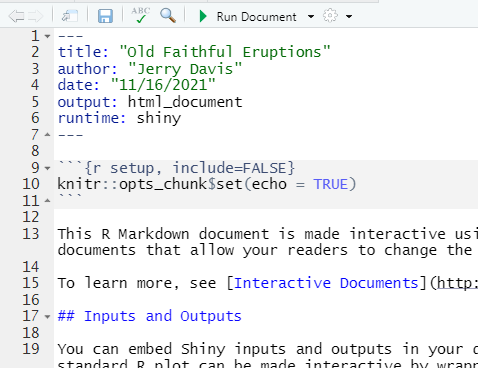Figure 16.2: Shiny Document Editor

The key to this document working in the shiny runtime environment is to make sure to specify this in the YAML header. At minimum, this header needs to include two settings:

---
output: html_document
runtime: shiny
---

Let’s start by going ahead and running it with the Run Document button, which due to the shiny runtime option replaces the Knit button we’d normally see in R Markdown.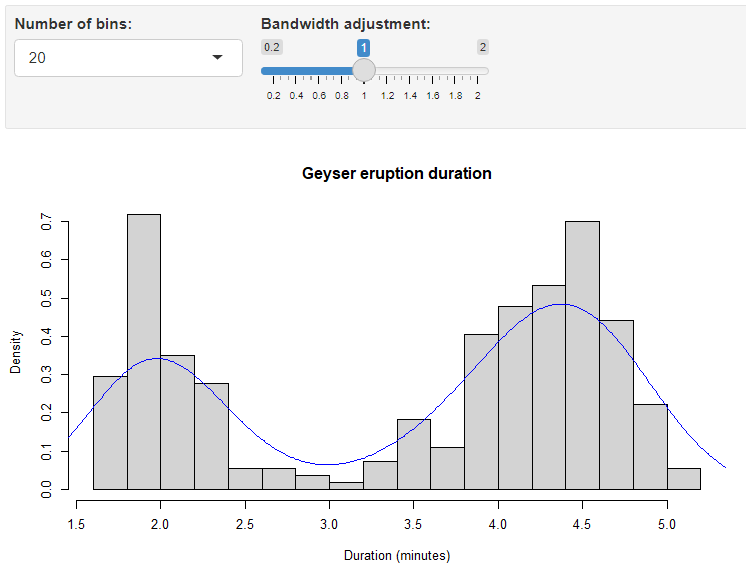Figure 16.3: Old Faithful geyser eruptions Shiny interface

So now we have a simple example to explore how it works. We’ll start by exploring its components, so keep this document open to try things out.

### 16.1.1 Input and output objects in the Old Faithful Eruptions document

In either markdown or app mode, we can create a variety of Shiny input widgets and output objects:

• Inputs are the interactive controls (widgets) that let the user change the resulting output.
• Outputs are the graphs, maps or tables, and are automatically updated whenever inputs change.

The code for the Old Faithful Eruptions document includes a couple of widget-controlled input settings to be used in a plot produced by renderPlot

• a selectInput that is used to set the breaks parameter for the hist function as a number of bins
• a sliderInput to set the adjust (bandwidth adjustment) parameter for the density plot

Note how the input variables are accessed by the output function as input$n_breaks and input$bw_adjust:

inputPanel(
selectInput("n_breaks", label = "Number of bins:",
choices = c(10, 20, 35, 50), selected = 20),

min = 0.2, max = 2, value = 1, step = 0.2)
)

This is then followed by the plot object:

renderPlot({
hist(faithful$eruptions, probability = TRUE, breaks = as.numeric(input$n_breaks),
xlab = "Duration (minutes)", main = "Geyser eruption duration")

dens <- density(faithful$eruptions, adjust = input$bw_adjust)
lines(dens, col = "blue")
})

This is then followed by an embedded shiny app, but we won’t look at it.

### 16.1.2 Input widgets

We’ll continue to explore the various input widgets and related outputs, and stick with R Markdown. You’ll still need to run the entire document, so you might want to make separate documents – just make sure they have the YAML header above. Go ahead and create a new Shiny document, the same as above, but create an empty document and then enter the YAML header and one code chunk to create a simple numericInput widget and print output.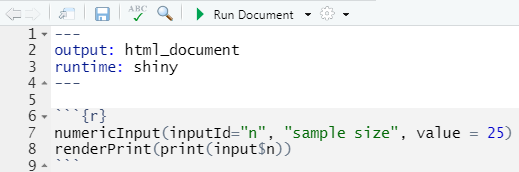Figure 16.4: numericInput and renderPrint code

numericInput(inputId="n", "sample size", value = 25)
renderPrint(print(input$n)) Once you save it, you’ll see the Run Document button if the YAML code is right. Go ahead and run it to see the input. It’s not very interesting, but you can see that the printed output changes with the input. Let’s add a slider: numericInput(inputId="n", "sample size", value = 25) renderPrint(print(input$n))
sliderInput(inputId="i", "input", min=1, max=30, value = 15)
renderPrint(print(input$i))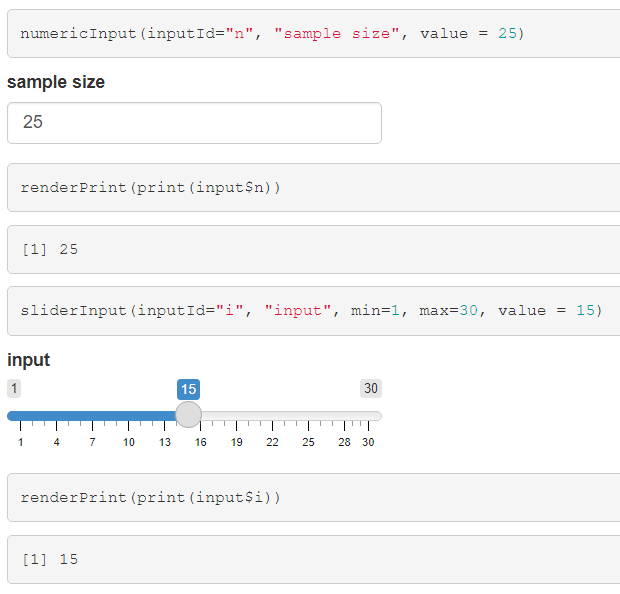Figure 16.5: Slider Input and Print We’ll explore some other input widgets and outputs. You can also see these on the Shiny cheatsheet … https://shiny.rstudio.com/images/shiny-cheatsheet.pdf … but just look at the part about the input and output objects. The cheatsheet is focused on creating Shiny Web Apps, so there’s a lot there about building that structure, with a user interface (ui) and server. We’ll get to those later. #### 16.1.2.1 A plot output The above widgets went to a rendered print output, but the same simple inputs can of course be used to create a plot: sliderInput(inputId="bins", "number of bins", min=1, max=30, value = 15) renderPlot(hist(rnorm(100), breaks=input$bins))Figure 16.6: Slider Input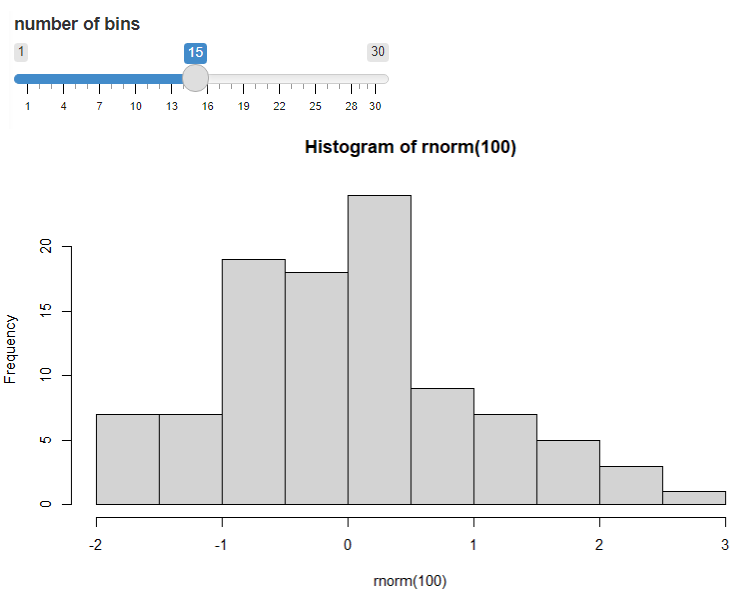Figure 16.7: Plot modified by input

### 16.1.3 Other input widgets

There are lots of other input widgets that are pretty easy to see how they apply based on the type of control we need to set:

• radioButtons() for choosing just one, and by default the first is chosen
radioButtons(inputId="which_one", label="Select:",
choices = c("Choice 1"="Choice1","Choice 2"="Choice2","Choice 3"="Choice3"))
renderPrint(print(input$which_one))• checkboxGroupInput() for choosing multiples checkboxGroupInput(inputId="which", label="Select:", choices = c("Choice 1"="Choice1","Choice 2"="Choice2","Choice 3"="Choice3")) renderPrint(print(input$which))• dateInput()
dateInput(inputId="date", label="Select date:")
renderPrint(print(input$date))Figure 16.8: Date input• textInput() textInput(inputId="text", label="Enter text:") renderPrint(print(input$text))Figure 16.9: Text input## 16.2 A Shiny app

The above input widgets and output objects we looked at in a Shiny document can also be built into a Shiny app, which has a place for inputs and outputs. The following simple Shiny app illustrates the basic elements:

• Optionally some code at the top to set things up, such as loading packages and setting up data.
• The ui section, which determines what the user will see, such as input widgets (like numericInput) and an area to plot things in, in this case plotOutput. In order to fit well on the page, the fluidPage function is used to hold it in; we could have also used inputPanel as we did earlier, but the result is not as well laid out.
• The server section, which uses two parameters (input, output) created in the user interface to:
• provide data from the input widget (in this case numericInput which creates input$n) such as parameter settings to functions (e.g. rnorm() here which needs a sample size provided by input$n) in the rendered output (of in this case hist)
• to render an output using a render___ method such as renderPlot which will run everything inside it (in this case a histogram)
• Providing that ui and server to the shinyApp function.
library(shiny)
ui <- fluidPage(
numericInput(input="n",
"Sample size", value=25),
plotOutput(outputId = "hist"))
server <- function(input, output) {
output$hist <- renderPlot({ hist(rnorm(input$n))
})
}
shinyApp(ui=ui,server=server)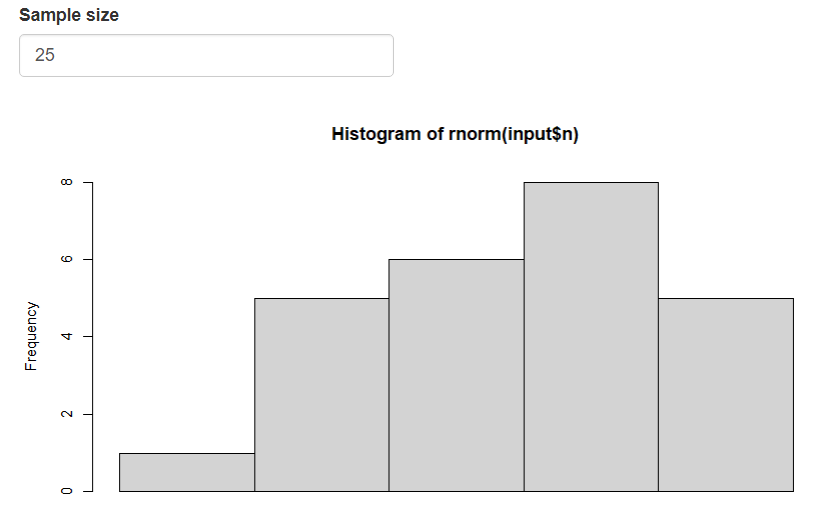Figure 16.10: Simple Inline app

This Shiny app is very short, which is useful in wrapping your head around these basic elements, which is important to understand. It can be run either as an inline app in R Markdown, in a standard R script (but all code has to be selected and then run), or as an app that can be published online.

We’ll go ahead and create a new Shiny Web App to run locally. You can either create a special type of RStudio project called Shiny Web Application, in a new or existing folder, or just create a Shiny Web App script as a file, as shown here. In either case, your app file should be named app.R.

• Use the file menu to create a new file, specifying Shiny Web App as the type, and it’ll end up being named app.R. (You can also name it something else, but this is the name you’ll need to use to create a fully functioning Shiny Web App.)
• Go with the defaults again, and you’ll end up with Shiny Web App version of the Old Faithful Eruptions document we created above, though a bit simpler, with only one input control and only a histogram.
• To run it, use the Run App button in the upper right of the code editor window.
• Have a look at what’s created in the app.R script.

Then either create a new blank R script, or edit this one and replace the old faithful code with the above even simpler code in the figure above. Note that to get the Run app button in the RStudio script editor window, the code just needs to include library(shiny) and be a complete app, with a ui and server section, and the shinyApp function at the end.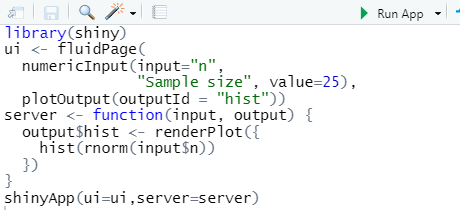Figure 16.11: Simple inline coding

### 16.2.1 A brief note on Reactivity

Since Shiny is an interactive environment, user operations are sensed as events, putting in motion a reaction of the program to that event. You can see that happening every time use adjust things in an input widget and the output changes in response. There’s a lot to reactivity, so please review the tutorial at https://shiny.rstudio.com/tutorial to gain a better understanding than we’ll be able to do here. We’ll look at some examples in the longer apps described below.

## 16.3 Shiny app I/O methods

One thing to note about how the Shiny web app works as opposed to just using the interactive methods in the Shiny Document we looked at earlier is the way the ui and server communicate about the plot creation: We didn’t use plotOutput in the I/O methods; we just used the input widgets to set parameters in the renderPlot.

Instead, this Shiny web app has the renderPlot in the server section, and a new function plotOutput is used to both specify the location of the plot and set outputId to be used as a variable to append to output$ in the server section. This can be a little confusing, so spend some time seeing how this works in the simple apps we just looked at, then start exploring other ones. The layout of the ui can make this even more confusing, as they’re set up in various ways. This isn’t surprising if you’ve spent much time in software development – the user interface is often the biggest challenge. Referring to the Outputs section of the cheat sheet, we can see that various render*() functions work with corresponding *Output() functions: • renderDataTable() works with dataTableOutput() • renderImage( ) works with imageOutput() • renderPlot( ) works with plotOutput() • renderPrint( ) works with verbatimTextOutput() • renderTable( ) works with tableOutput() • renderText( ) works with textOutput() • renderUI( ) works with uiOutput() and htmlOutput() And there are others. For instance, as we’ll see in the next document: • renderLeaflet( ) works with leafletOutput() ### 16.3.1 Data Tables: renderDataTable() and dataTableOutput() library(shiny); library(tidyverse) ui <- fluidPage( numericInput(input="n", "Sample size", value=25), dataTableOutput(outputId = "varTable")) server <- function(input, output) { output$varTable <- renderDataTable(tibble(a=rnorm(input$n), b=rnorm(input$n)))}
shinyApp(ui=ui,server=server)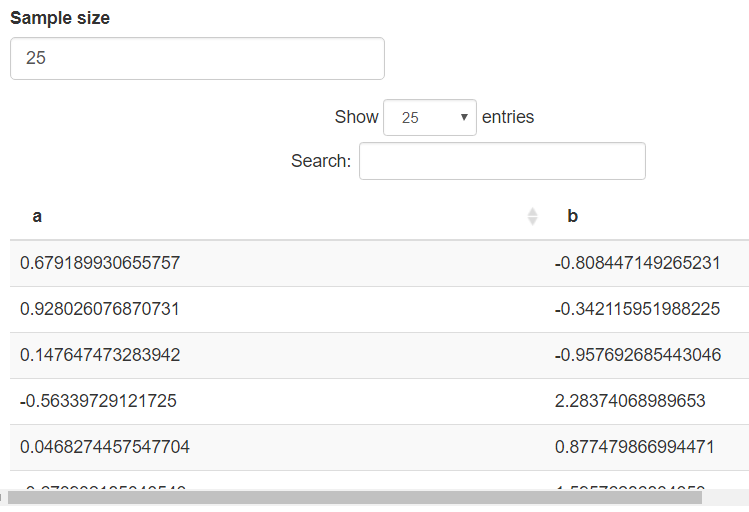Figure 16.12: Rendered data table

### 16.3.2 Text as Character: renderPrint() and verbatimTextOutput()

These functions produce a mono-spaced-font output like a character variable.

library(shiny); library(tidyverse)
ui <- fluidPage(
textInput(inputId="monotxt", label="Enter text:", value="Some text"),
verbatimTextOutput(outputId = "txt"))
server <- function(input, output) {
output$txt <- renderPrint(print(input$monotxt))}
shinyApp(ui=ui,server=server)Figure 16.13: Text entry

### 16.3.3 Formatted text: renderText() and textOutput()

library(shiny); library(tidyverse)
ui <- fluidPage(
textInput(inputId="text", label="Enter text:", value="Some text"),
textOutput(outputId = "prn"))
server <- function(input, output) {
output$prn <- renderText(print(input$text))}
shinyApp(ui=ui,server=server)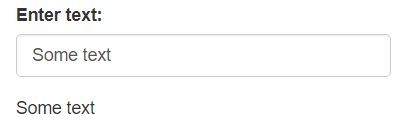### 16.3.4 Plots: renderPlot() and plotOutput()

library(shiny); library(tidyverse)
ui <- fluidPage(
sliderInput(inputId="i", "input", min=1, max=30, value = 15),
textInput(inputId="title", label="Enter title:", value="Tukey boxplot"),
plotOutput(outputId = "Tukey"))
server <- function(input, output) {
output$Tukey <- renderPlot(boxplot(rnorm(input$i),main=input$title))} shinyApp(ui=ui,server=server)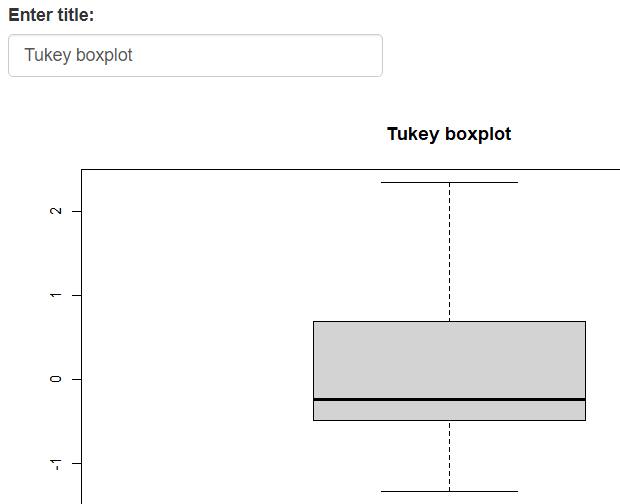Figure 16.14: Rendered box plot ## 16.4 Shiny app in a package A Shiny App project folder can also be stored in the extdata folder of a data package. Here’s a sierra app accessed this way, which you can run from RStudio, either pasted into the console or saved in a script and run by marking the text and running. The Run app button won’t appear even from a saved script, because what you’re editing is not a full Shiny app script; it just calls one. shiny::shinyAppDir(system.file("extdata","sierra",package="igisci"), options = list(width = "100%", height = 700)) Note the use of system.file to provide the app folder location to shinyAppDir. You can find this sierra folder in the igisci extdata folder, so as long as you have the data package installed, it should run. And the igisci package has a function sierra() that simply runs the above so that’s all you have to enter if you have the library in effect with library(igisci), and you’ll see it appear in a new window.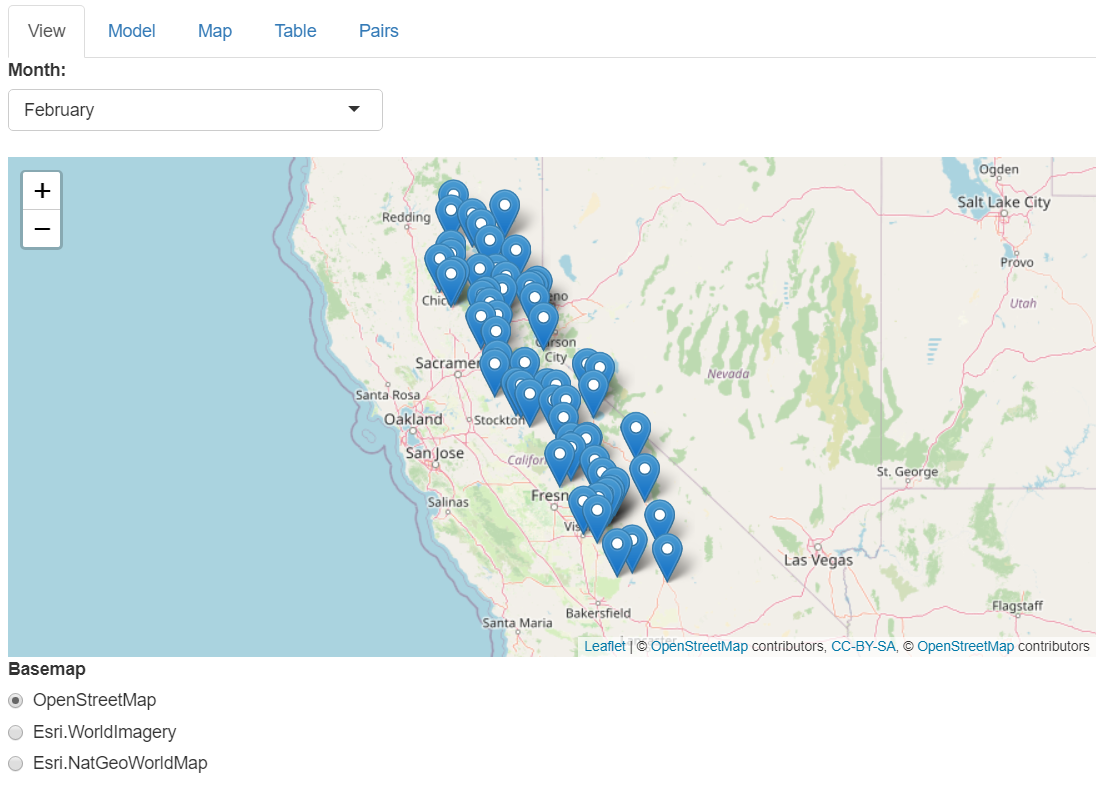Figure 16.15: Shiny app of Sierra climate data, with multiple tabs available We’ll look at this app in detail next, so you should have it open in another window, using the above method, or simply by running: igisc::sierra() ## 16.5 Components of a Shiny app (sierra) Lets break down the components of the sierra Shiny app to see how a tabsetPanel Shiny app works. A review of the cheat sheet will show you that this is just one of a variety of Shiny app ui structures. We’ll also see how reactive elements work in the server section. ### 16.5.1 Initial data setup Most Shiny apps are going to need some initial code that sets up the data. I sometimes use the source method to call this code. We won’t look at this now, but it’s provided here to be able to identify where data in the main Shiny app code goes. library(shiny); library(sf); library(leaflet); library(rgdal) library(tidyverse); library(terra) library(igisci) sierraAllMonths <- read_csv(ex("sierra/Sierra2LassenData.csv")) %>% filter(MLY_PRCP_N >= 0) %>% filter(MLY_TAVG_N >= -100) %>% rename(PRECIPITATION = MLY_PRCP_N, TEMPERATURE = MLY_TAVG_N) %>% mutate(STATION = str_sub(STATION_NA, end=str_length(STATION_NA)-6)) sierraJan <- sierraAllMonths %>% # to create an initial model and variable name symbols sample_n(0) %>% # sample_n(0) samples zero, just gets the variable names dplyr::select(LATITUDE, LONGITUDE, ELEVATION, TEMPERATURE, PRECIPITATION) sierraVars <- sierraJan %>% # Builds list of variables for map mutate(RESIDUAL = numeric(), PREDICTION = numeric()) %>% dplyr::select(ELEVATION, TEMPERATURE, PRECIPITATION, RESIDUAL, PREDICTION) # Create basemap, using the weather station points to set the bounding dimensions co <- CA_counties ct <- st_read(ex("sierra/CA_places.shp")) ct$AREANAME_pad <- paste0(str_replace_all(ct$AREANAME, '[A-Za-z]',' '), ct$AREANAME)
hillsh <- rast(ex("CA/ca_hillsh_WGS84.tif"))
hillshptsT <- as.points(hillsh)
hillshpts <- st_as_sf(hillshptsT)
CAbasemap <- ggplot() +
geom_sf(data = hillshpts, aes(col=ca_hillsh_WGS84)) + guides(color = F) +
geom_sf(data = co, fill = NA) +
scale_color_gradient(low = "#606060", high = "#FFFFFF") +
labs(x='',y='')
spdftemp <- st_as_sf(sierraAllMonths, coords = c("LONGITUDE","LATITUDE"), crs=4326)
bounds <- st_bbox(spdftemp)
sierrabasemap <- CAbasemap +
geom_sf(data=ct) +
geom_sf_text(mapping = aes(label=AREANAME_pad), data=ct, size = 3,
nudge_x = 0.1, nudge_y = 0.1) +
coord_sf(xlim = c(bounds, bounds), ylim = c(bounds,bounds))

# Function used by pairs plot:
panel.cor <- function(x, y, digits = 2, prefix = "", cex.cor, ...)
{
usr <- par("usr"); on.exit(par(usr))
par(usr = c(0, 1, 0, 1))
r <- abs(cor(x, y))
txt <- format(c(r, 0.123456789), digits = digits)
txt <- paste0(prefix, txt)
if(missing(cex.cor)) cex.cor <- 0.8/strwidth(txt)
text(0.5, 0.5, txt, cex = cex.cor * r)
}

### 16.5.2 The ui section, with a tabsetPanel structure

In this section, we’re setting up the tabsetPanel structure, allowing the user to select whether to look at the various outputs:

• View: A leaflet map of the Sierra stations, and an ability to choose which month to process, and radio buttons to choose a basemap. The month choice structure took a bit to figure out; note the named indices.
• Model: Scatter plot and trend line of a linear model, with the ability to change the x and y variables.
• Map: Map of the variables in the data, or the regression or residuals. Input to choose which to display.
• Table: Table of the data for the chosen month. No inputs.
• Pairs: A pairs plot of the data, for the chosen month. No inputs.
ui <- fluidPage(title = "Sierra Climate",
tabsetPanel(
tabPanel(title = "View",
selectInput("month", "Month:",
c("January"=1, "February"=2, "March"=3,
"April"=4,   "May"=5,      "June"=6,
"July"=7,    "August"=8,   "September"=9,
"October"=10,"November"=11,"December"=12)),
leafletOutput("view"),
radioButtons(inputId = "LeafletBasemap", label = "Basemap",
choices = c("OpenStreetMap" = "open",
"Esri.WorldImagery" = "imagery",
"Esri.NatGeoWorldMap" = "natgeo"),
selected = "open")),
tabPanel(title = "Model",
plotOutput("scatterplot"),
varSelectInput("xvar", "X Variable:", data=sierraJan,
selected="ELEVATION"),
varSelectInput("yvar", "Y Variable:", data=sierraJan,
selected="TEMPERATURE"),
verbatimTextOutput("model")),
tabPanel(title = "Map",
plotOutput("map"),
varSelectInput("var", "Variable:", data=sierraVars,
selected="TEMPERATURE")),
tabPanel(title = "Table",
textOutput("eqntext"),
tableOutput("table")),
tabPanel(title = "Pairs",
textOutput("monthTitle4Pairs"),
plotOutput("pairsplot"))
)
)

### 16.5.3 The server section, including reactive elements

This section starts with a series of specifically reactive functions using the reactive function. These are functions that are used in the main output (or in other reactive functions) that change the data. Here we see the following reactive functions:

• mod() : reads the input$xvar and input$yvar and creates a linear model
• sierraMonth(): reads the input$month and filters the month and selects all the relevant variables • sierradf(): runs mod() and assigns RESIDUAL and PREDICTION variables • sierraSp(): responds to sierradf() by then creating an sf • eqn(): responds to mod() by changing the model character string to put on a map server <- function(input, output) { mod <- reactive({ lm(as.formula(paste(input$yvar, '~', input$xvar)), data=sierraMonth()) }) sierraMonth <- reactive({ monthsel <- 201000 + as.numeric(input$month)
sierraAllMonths %>%
filter(DATE == monthsel) %>%
dplyr::select(STATION, ELEVATION, LATITUDE, LONGITUDE, TEMPERATURE, PRECIPITATION)
})
sierraMonth() %>%
mutate(RESIDUAL = resid(mod()), PREDICTION = predict(mod()))
})
sierraSp <- reactive(st_as_sf(sierradf(), coords = c("LONGITUDE","LATITUDE"), crs=4326))

eqn <- reactive({
cc = mod()$coefficient paste(input$yvar, " =", paste(round(cc,2), "+", paste(round(cc[-1], digits=3),
sep="*", collapse=" + ",
paste(input$xvar)))) }) Then the outputs, each with a render____ function: • output$view: a Leaflet map for the View tab
• output$map: a ggplot map for the Map tab • output$scatterplot: a scatter plot and trend line for the Model tab
• output$model: a summary of the lm • output$eqntext: information on the model for the Table tab
• output$monthTitle4Model: a title for the Model tab • output$monthTitle4Pairs: a title for the Pairs tab
• output$table: the table for the Table tab • output$pairsplot: the pairs plot for the Pairs tab
  output$view <- renderLeaflet({ providerTiles <- providers$OpenStreetMap
if(input$LeafletBasemap == "imagery") {providerTiles <- providers$Esri.WorldImagery}
if(input$LeafletBasemap == "natgeo") {providerTiles <- providers$Esri.NatGeoWorldMap}
popup = ~str_c(ELEVATION,"m ", month.name[as.numeric(input$month)], ": ", TEMPERATURE, "°C ", PRECIPITATION, "mm"), label = ~STATION) }) output$map <- renderPlot({
subTitle <- ""
if((input$var == "RESIDUAL")|(input$var == "PREDICTION")){
subTitle <- eqn()}
v <- get(paste(input$var), pos=sierradf()) # just to be able to use the vector sierrabasemap + geom_sf(mapping = aes(color = !!input$var), data=sierraSp(), size=4) +
coord_sf(xlim = c(bounds, bounds), ylim = c(bounds,bounds))  +
labs(title=paste(month.name[as.numeric(input$month)], input$var),
subtitle=subTitle) + theme(legend.position = c(0.8, 0.85))
})
output$scatterplot <- renderPlot({ ggplot(data = sierradf()) + geom_point(mapping = aes(x = !!input$xvar, y = !!input$yvar)) + geom_smooth(mapping = aes(x = !!input$xvar, y = !!input$yvar), method="lm") + labs(title=month.name[as.numeric(input$month)])
})
output$model <- renderPrint({ print(eqn()) summary(mod()) }) output$eqntext <- renderText(paste(month.name[as.numeric(input$month)], "data. Residual and Prediction based on linear model: ", eqn())) output$monthTitle4Model <- renderText(month.name[as.numeric(input$month)]) output$monthTitle4Pairs <- renderText(month.name[as.numeric(input$month)]) output$table <- renderTable(sierradf())
output$pairsplot <- renderPlot({ sierradf() %>% dplyr::select(LATITUDE,LATITUDE,LONGITUDE,ELEVATION,TEMPERATURE,PRECIPITATION) %>% pairs(upper.panel = panel.cor) })} ### 16.5.4 Calling shinyApp with the ui and server function results And finally calling both ui and server functions with shinyApp: shinyApp(ui = ui, server = server) ## 16.6 A MODIS fire app with web scraping and observe with leafletProxy We’ll now look at another app, one that uses web scraping and an observe function that together with leafletProxy allows the map to maintain its scaling when we change the data. The MODIS satellite sensor includes a fire detection layer that the USFS hosts in a way that can be accessed by (some pretty primitive) web scraping, which you can see in the code. This R Markdown document is made interactive using Shiny, and employs an Inline Application with the complete Shiny app code included. To learn more about the MODIS product from NASA, see https://modis.gsfc.nasa.gov/data/dataprod/mod14.php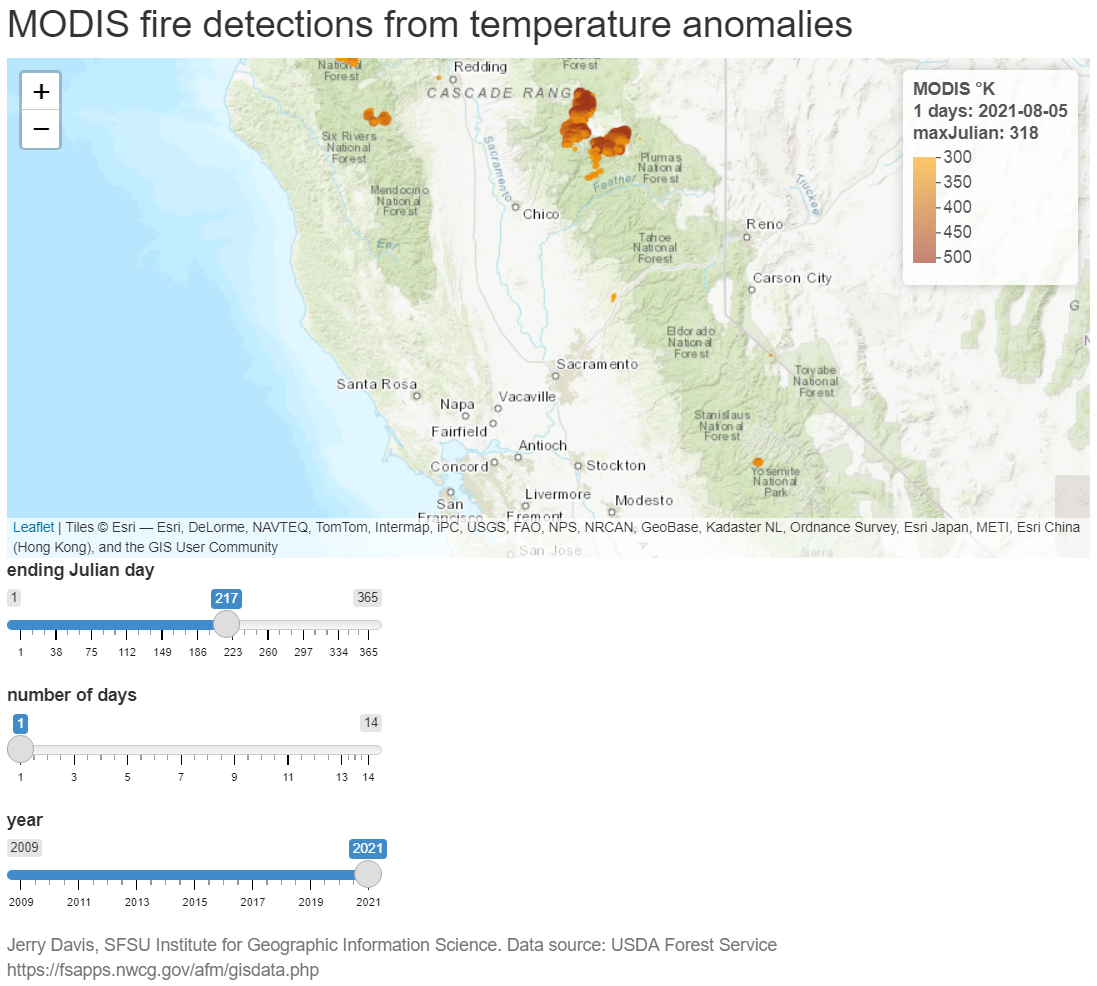Figure 16.16: MODIS fire detection Shiny app The MODISfire app.R script is also in the data package, in the MODISfire folder, and can be run with igisc::MODISfire(), which simply runs the following installed as a function with no input parameters, creating a separate app window. shiny::shinyAppDir( system.file("extdata","MODISfire",package="igisci"), options = list(width = "100%", height = 700)) Note that it takes a while for the map to appear because the data is being downloaded and processed. Every time you change to a new year, there is also a delay as new data is downloaded and processed. The code shown can also be used to create a Shiny web app by saving it as app.R in an RStudio Shiny app project (or just copying the app.R file from the MODISfire folder in extdata). It will create a "MODISdata" folder to hold data it downloads, to keep the location with Shiny Rmd files uncluttered with the downloaded shapefile data. ### 16.6.1 Setup code Note that we’ll create a folder to hold data we download, and make it the working directory. library(sf); library(leaflet); library(tidyverse); library(lubridate) library(shiny); library(here) dataPath <- paste(here::here(),"/MODISdata",sep="") if (!file.exists(dataPath)){dir.create(dataPath)} setwd(dataPath) pal <- colorNumeric(c("orange", "firebrick4"),domain=300:550) ### 16.6.2 ui In terms of complexity of inputs, this app is pretty straightforward – the fluidPage is pretty standard for the ui section. ui <- fluidPage( titlePanel("MODIS fire detections from temperature anomalies"), leafletOutput("view"), sliderInput(inputId = "end_jday", label = "ending Julian day", value = yday(now()), min = 1, max = 365, step = 1), sliderInput(inputId = "numdays", label = "number of days", value = 1, min = 1, max = 14, step = 1), sliderInput(inputId = "year", label = "year", value = year(now()), min = 2009, max = year(now()), step = 1, sep = ""), helpText(paste("Jerry Davis, SFSU Institute for Geographic Information Science.", "Data source: USDA Forest Service https://fsapps.nwcg.gov/afm/gisdata.php")) ) ### 16.6.3 Using observe and leafletProxy to allow changing the date while retaining the map zoom In the server section, we mostly just have the leaflet map, but we need to build in code to do the web scraping and allow changing the date while retaining the zoom. Web scraping was used to download data for a given year from the USFS. Hopefully their file naming convention stays the way it is; you can see how the shapefile name string is built with wildcard dots for characters. observe and leafletProxy: Note that in the renderLeaflet section, only the year is reactive so this runs only initially or when the year changes. The observe function doesn’t read the data anew, but just changes some parameters about the map. The trick to getting this to work was then to use leafletProxy to make changes to the map without recreating it. Before I figured this out, the map would start over at its beginning point and you couldn’t then change the date for an area you just zoomed to. This took a while to figure out… server <- function(input, output, session) { output$view <- renderLeaflet({  # Only year is reactive, so only runs initially/year change
yrst <- as.character(input$year) txt <- read_file(str_c("https://fsapps.nwcg.gov/afm/data/fireptdata/modisfire_", yrst,"_conus.htm")) shpPath <- str_extract(txt, "https://fsapps.nwcg.gov/afm/data/fireptdata/modis_fire_.........conus_shapefile.zip") shpZip <- str_extract(shpPath, "modis_fire_.........conus_shapefile.zip") MODISfile <- str_c(dataPath,"/",str_extract(shpZip, "modis_fire_.........conus"),".shp") if(yrst == as.character(year(now())) | !file.exists(MODISfile)) { shpZipPath <- str_c(dataPath, "/",shpZip) download.file(shpPath, shpZipPath) unzip(shpZipPath, exdir=dataPath) } fires <<- st_read(MODISfile) leaflet() %>% addProviderTiles(providers$Esri.WorldTopoMap) %>%
fitBounds(-123,37,-120,39)
})
observe({                   # Allows the map to retain its location and zoom
numdays <- input$numdays; end_jday <- input$end_jday
fireFilt <- filter(fires,between(JULIAN, end_jday - numdays, end_jday))
yrst <- as.character(input$year) dat <- as.Date(end_jday-1, origin=str_c(yrst,"-01-01")) # Julian day fix leafletProxy("view", data = fireFilt) %>% clearMarkers() %>% addCircleMarkers( radius = ~(TEMP-250)/50, # scales 300-500 from 1:5 color = ~pal(TEMP), stroke = FALSE, fillOpacity = 0.8) %>% clearControls() %>% # clears the legend addLegend("topright", pal=pal, values=~TEMP, opacity=0.6, title=str_c("MODIS °K</br>",numdays, " days: ", dat,"</br>maxJulian: ",as.character(max(fires$JULIAN))))
})
}
shinyApp(ui = ui, server = server, options = list(width = "100%", height = 800))